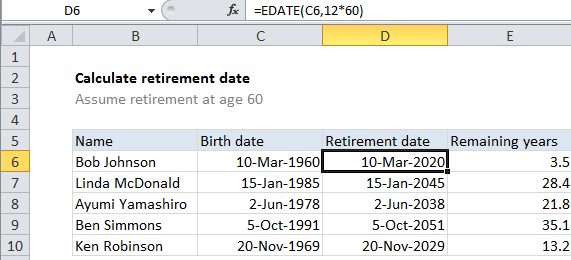## Excel Office

Excel How Tos, Tutorials, Tips & Tricks, Shortcuts

# Calculate retirement date in Excel

If you need to calculate retirement date in Excel, then this tutorials is for you.

To calculate a retirement date based on a birth date, you can use the EDATE function. See example below;

## Formula

`=EDATE(A1,12*60)`## Explanation

In the example shown, the formula in D6 is:

`=EDATE(C6,12*60)`

### How this formula works

The EDATE function is fully automatic, and will return a date xx months in the future or past, when given a date and the number of months to traverse.

Also See:   How to get year from date in Excel

In this case, we want a date 60 years in the future, starting with a birthdate, so we can write a formula like this for the data in the example:

`=EDATE(C6,12*60)`

The date comes from the birth dates in column C. For months, we need the equivalent of 60 years in months. Since you probably don’t know how many months are in 60 years, a nice way to do this is to embed the math for that calculation directly in the formula:

`12*60`

Excel will resolve this to 720, then feed that into EDATE for months. Embedding calculations this way can can help make the assumptions and purpose of an argument clear.

Also See:   How to determine year is a leap year in Excel

Note: EDATE returns a date in Excel’s serial number format, so make sure you apply date formatting.

### Remaining years

The formula used to get remaining years in column E is:

`=YEARFRAC(TODAY(),D6)`

You can use this same approach to calculate age from birthdate.

### Just the year, thanks

What if you just want to know the year of retirement? In that case, you can format the date returned by EDATE with the custom number format “yyyy”, or, if you really want only the year, you can wrap the result in the YEAR function like this:

`=YEAR(EDATE(A1,12*60))`
Also See:   How to Calculate Age in Excel